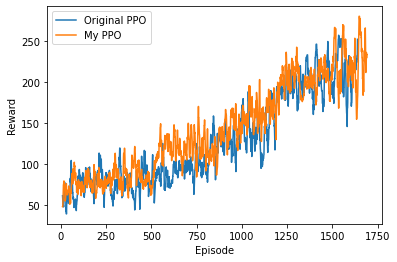# [PYTHON] I want to use a network defined by myself in PPO2 of Stable Baselines

In Until you clear Super Mario Bros. 1-1 using Stable Baselines, you learned using PPO2 provided in Stable Baselines.

PPO2 just gives the name'CNNPolicy', and I'm not sure exactly what kind of architecture network is used (although there is a description that it conforms to the original PPO paper). Also, I didn't know what to do if I wanted to modify the network, so I followed Original Code.

#### `ppo2.py`

``````
class PPO2(ActorCriticRLModel):
def __init__(self, policy, env, gamma=0.99, n_steps=128, ent_coef=0.01, learning_rate=2.5e-4, vf_coef=0.5,
max_grad_norm=0.5, lam=0.95, nminibatches=4, noptepochs=4, cliprange=0.2, cliprange_vf=None,
verbose=0, tensorboard_log=None, _init_setup_model=True, policy_kwargs=None,
full_tensorboard_log=False, seed=None, n_cpu_tf_sess=None):
super().__init__(policy=policy, env=env, verbose=verbose, requires_vec_env=True,
_init_setup_model=_init_setup_model, policy_kwargs=policy_kwargs,
seed=seed, n_cpu_tf_sess=n_cpu_tf_sess)

``````

Keywords such as'CNNPolicy' correspond to this argument policy. However, it is not explicitly used within the init of this class, only within the parent class init.

Let's go see the parent class ActorCriticRLModel. It is located in stable-baselines/stable_baselines/common/base_class.py.

#### `base_class.py`

``````
class ActorCriticRLModel(BaseRLModel):
def __init__(self, policy, env, _init_setup_model, verbose=0, policy_base=ActorCriticPolicy,
requires_vec_env=False, policy_kwargs=None, seed=None, n_cpu_tf_sess=None):
super(ActorCriticRLModel, self).__init__(policy, env, verbose=verbose, requires_vec_env=requires_vec_env,
policy_base=policy_base, policy_kwargs=policy_kwargs,
seed=seed, n_cpu_tf_sess=n_cpu_tf_sess)
``````

Again, it's used in the parent class init. The parent class BaseRLModel is defined as an Abstract Class in the same file.

#### `base_class.py`

``````
class BaseRLModel(ABC):
def __init__(self, policy, env, verbose=0, *, requires_vec_env, policy_base,
policy_kwargs=None, seed=None, n_cpu_tf_sess=None):
if isinstance(policy, str) and policy_base is not None:
self.policy = get_policy_from_name(policy_base, policy)
else:
self.policy = policy
``````

Apparently it gets it with a function called get_policy_from_name. get_policy_from_name is defined in stable-baselines/stable_baselines/common/policies.py.

#### `policies.py`

``````
def get_policy_from_name(base_policy_type, name):
if base_policy_type not in _policy_registry:
raise ValueError("Error: the policy type {} is not registered!".format(base_policy_type))
if name not in _policy_registry[base_policy_type]:
raise ValueError("Error: unknown policy type {}, the only registed policy type are: {}!"
.format(name, list(_policy_registry[base_policy_type].keys())))
return _policy_registry[base_policy_type][name]
``````

We use a dictionary called policy_registry to call the class corresponding to policy. policy_registry is written just above it in the same file.

#### `policies.py`

``````
_policy_registry = {
ActorCriticPolicy: {
"CnnPolicy": CnnPolicy,
"CnnLstmPolicy": CnnLstmPolicy,
"CnnLnLstmPolicy": CnnLnLstmPolicy,
"MlpPolicy": MlpPolicy,
"MlpLstmPolicy": MlpLstmPolicy,
"MlpLnLstmPolicy": MlpLnLstmPolicy,
}
}
``````

I want to know the contents of CnnPolicy here, so I will go to see it. This is also defined in the same file.

#### `policies.py`

``````
class CnnPolicy(FeedForwardPolicy):
"""
Policy object that implements actor critic, using a CNN (the nature CNN)
:param sess: (TensorFlow session) The current TensorFlow session
:param ob_space: (Gym Space) The observation space of the environment
:param ac_space: (Gym Space) The action space of the environment
:param n_env: (int) The number of environments to run
:param n_steps: (int) The number of steps to run for each environment
:param n_batch: (int) The number of batch to run (n_envs * n_steps)
:param reuse: (bool) If the policy is reusable or not
:param _kwargs: (dict) Extra keyword arguments for the nature CNN feature extraction
"""

def __init__(self, sess, ob_space, ac_space, n_env, n_steps, n_batch, reuse=False, **_kwargs):
super(CnnPolicy, self).__init__(sess, ob_space, ac_space, n_env, n_steps, n_batch, reuse,
feature_extraction="cnn", **_kwargs)
``````

Since it seems that the entity is not written here, look at the FeedForwardPolicy of the parent class.

#### `policies.py`

``````
class FeedForwardPolicy(ActorCriticPolicy):

def __init__(self, sess, ob_space, ac_space, n_env, n_steps, n_batch, reuse=False, layers=None, net_arch=None,
act_fun=tf.tanh, cnn_extractor=nature_cnn, feature_extraction="cnn", **kwargs):
super(FeedForwardPolicy, self).__init__(sess, ob_space, ac_space, n_env, n_steps, n_batch, reuse=reuse,
scale=(feature_extraction == "cnn"))

self._kwargs_check(feature_extraction, kwargs)

if layers is not None:
warnings.warn("Usage of the `layers` parameter is deprecated! Use net_arch instead "
"(it has a different semantics though).", DeprecationWarning)
if net_arch is not None:
warnings.warn("The new `net_arch` parameter overrides the deprecated `layers` parameter!",
DeprecationWarning)

if net_arch is None:
if layers is None:
layers = [64, 64]
net_arch = [dict(vf=layers, pi=layers)]

with tf.variable_scope("model", reuse=reuse):
if feature_extraction == "cnn":
pi_latent = vf_latent = cnn_extractor(self.processed_obs, **kwargs)
else:
pi_latent, vf_latent = mlp_extractor(tf.layers.flatten(self.processed_obs), net_arch, act_fun)

self._value_fn = linear(vf_latent, 'vf', 1)

self._proba_distribution, self._policy, self.q_value = \
self.pdtype.proba_distribution_from_latent(pi_latent, vf_latent, init_scale=0.01)

self._setup_init()

def step(self, obs, state=None, mask=None, deterministic=False):
if deterministic:
action, value, neglogp = self.sess.run([self.deterministic_action, self.value_flat, self.neglogp],
{self.obs_ph: obs})
else:
action, value, neglogp = self.sess.run([self.action, self.value_flat, self.neglogp],
{self.obs_ph: obs})
return action, value, self.initial_state, neglogp

def proba_step(self, obs, state=None, mask=None):
return self.sess.run(self.policy_proba, {self.obs_ph: obs})

def value(self, obs, state=None, mask=None):
return self.sess.run(self.value_flat, {self.obs_ph: obs})
``````

I finally found something like that. The format is such that the features extracted using the feature extraction network are flowed into the value estimation network and the action probability output network. The following parts define the network for feature extraction.

#### `policies.py`

``````
if feature_extraction == "cnn":
pi_latent = vf_latent = cnn_extractor(self.processed_obs, **kwargs)
else:
pi_latent, vf_latent = mlp_extractor(tf.layers.flatten(self.processed_obs), net_arch, act_fun)
``````

The cnn_extractor function is an argument, which defaults to nature_cnn. The nature_cnn function is also defined in the same file.

#### `policies.py`

``````
def nature_cnn(scaled_images, **kwargs):
"""
CNN from Nature paper.
:param scaled_images: (TensorFlow Tensor) Image input placeholder
:param kwargs: (dict) Extra keywords parameters for the convolutional layers of the CNN
:return: (TensorFlow Tensor) The CNN output layer
"""
activ = tf.nn.relu
layer_1 = activ(conv(scaled_images, 'c1', n_filters=32, filter_size=8, stride=4, init_scale=np.sqrt(2), **kwargs))
layer_2 = activ(conv(layer_1, 'c2', n_filters=64, filter_size=4, stride=2, init_scale=np.sqrt(2), **kwargs))
layer_3 = activ(conv(layer_2, 'c3', n_filters=64, filter_size=3, stride=1, init_scale=np.sqrt(2), **kwargs))
layer_3 = conv_to_fc(layer_3)
return activ(linear(layer_3, 'fc1', n_hidden=512, init_scale=np.sqrt(2)))
``````

I finally found it. Instead of this, it seems that you should create a function that returns the network structure you want to use.

That's why I tried Mario 1-1 using a network with Self-Attention.However, there was no particular improvement in learning speed.